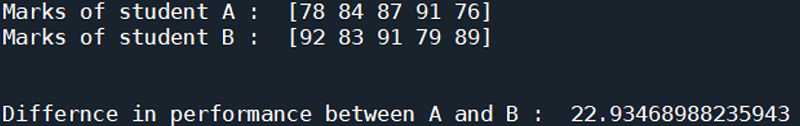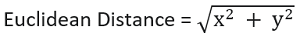# Euclidean Distance Example | Linear Algebra using Python

Linear Algebra using Python | Euclidean Distance Example: Here, we are going to learn about the euclidean distance example and its implementation in Python.
Submitted by Anuj Singh, on June 20, 2020

Prerequisite:In mathematics, the Euclidean distance is an ordinary straight-line distance between two points in Euclidean space or general n-dimensional space. If two students are having their marks of all five subjects represented in a vector (different vector for each student), we can use the Euclidean Distance to quantify the difference between the students' performance.x, y are the vectors in representing marks of student A and student B respectively.

## Python code for Euclidean distance example

```# Linear Algebra Learning Sequence
# Euclidean Distance Example

import numpy as np

a = np.array([78, 84, 87, 91, 76])
b = np.array([92, 83, 91, 79, 89])

# Finding the euclidean distance
dis = np.linalg.norm(a-b)

print('Marks of student A : ', a)
print('Marks of student B : ', b)

print('\n\nDiffernce in performance between A and B : ', dis)
```

Output:

```A :  [ 2  4  8  9 -6]
B :  [2 3 1 7 8]

Cosine Similarity between A and B :  0.2440982792118955
```

Preparation

What's New

Top Interview Coding Problems/Challenges!